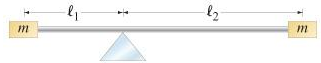# Problem: Two blocks, each of mass m, are attached to the ends of a massless rod which pivots as shown in the figure. Initially the rod is held in the horizontal position and then released.Calculate the magnitude of the net torque on this system when it is first released.Express your answer in terms of the variables L1, L2 , m, and appropriate constants.

###### FREE Expert Solution

Torque:

$\overline{){\mathbf{\tau }}{\mathbf{=}}{\mathbf{r}}{\mathbf{·}}{\mathbf{F}}}$

Take clockwise direction to be negative.

80% (258 ratings)###### Problem DetailsTwo blocks, each of mass m, are attached to the ends of a massless rod which pivots as shown in the figure. Initially the rod is held in the horizontal position and then released.

Calculate the magnitude of the net torque on this system when it is first released.
Express your answer in terms of the variables L1, L2 , m, and appropriate constants.